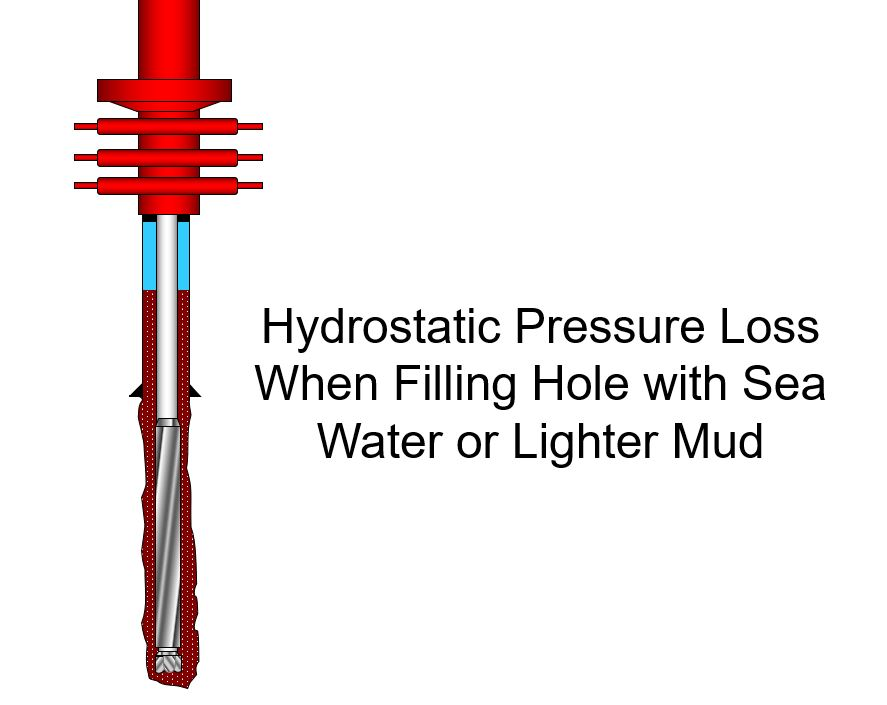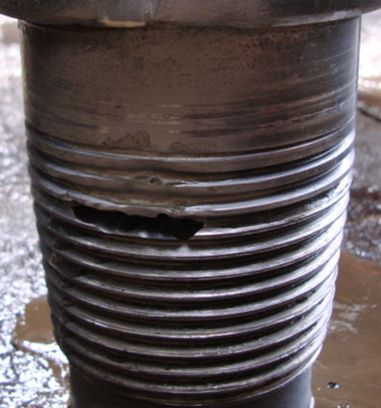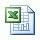## Loss of Hydrostatic Pressure When Filling Hole with Water or Lighter MudIn case of totally lost return, the annulus must be fully filled with fluid, normally water, as fast as we can. Water filled in annulus causes loss of hydrostatic pressure in the wellbore. This article demonstrates how to determine hydrostatic pressure reduction when water or other light fluid is used to fully fill the hole.Note: This calculation is based on if the float is in the drill string. Therefore, there is no way that fluid from annulus can come into the string.

There are two main concepts, annular capacity and hydrostatic pressure, applied to determine loss of hydrostatic pressure.

## Oilfield Unit

Height of feet of water in annulus

Height of water added  (ft)= water added in bbl ÷ annular capacity in bbl/ft

Bottomhole (BHP) pressure reduction

BHP decrease in psi = (current mud weight in ppg – weight of water in ppg) × 0.052 × (ft of water added)

Note: In order to calculate bottom hole pressure reduction, we assume the column of water in annulus is true vertical depth (TVD). This calculation may not be accurate if the well has high angle so you need to determine the actual TVD from directional survey data. However, this method will be the worst case scenario of pressure reduction.

Equivalent Mud Weight at TD

EMW in ppg = current mud weight in ppg – (BHP decrease in psi ÷ 0.052 ÷ TVD ft of hole)

Example: Determine bottom hole pressure loss and equivalent mud weight at TD due to filling up water into annulus.

Mud weight = 13.0 ppg
Water added = 140 bbl required to fill annulus
Weight of water = 8.6 ppg **
Annular capacity = 0.1422 bbl/ft
Hole TVD = 6,000 ft

Number of feet of water in annulus

Feet of water in annulus = 140 bbl ÷ 0.1422 bbl/ft

Feet = 984.5 ft

Bottomhole (BHP) pressure reduction

BHP reduction = (13.0 ppg – 8.6 ppg) × 0.052 × 984.5 ft

BHP reduction = 225.3 psi

Equivalent mud weight at TD

EMW in ppg = 13.0 – (225.3 psi ÷ (0.052× 6,000 ft))

EMW = 12.3 ppg

## Metric Unit

Height of water in annulus

Height of water added  (m) = water added in m3 ÷ annular capacity in m3/m

Bottomhole (BHP) pressure reduction

BHP decrease in psi = (current mud weight in kg/m3 – weight of water in kg/m3) × 0.00981 × (m of water added)

Equivalent Mud Weight at TD

EMW in ppg = current mud weight in kg/m3 – (BHP decrease in KPa ÷ 0.00981 ÷ TVD m of hole)

Example: Determine bottom hole pressure loss and equivalent mud weight at TD due to filling up water into annulus.

Mud weight = 1550 kg/m3
Weight of water = 1030 kg/m3
Annular capacity = 0.07417 m/m
Hole TVD = 2000 m

Height of water in annulus

Height of water in annulus= 20 m3 ÷ 0.07417 m3/m

Height of water in annulus = 270 m

Bottomhole (BHP) Pressure Reduction

BHP reduction = (1550 kg/m3 – 1030 kg/m3) × 0.00981 × 270 m

BHP reduction = 1,375 KPa

Equivalent mud weight at TD

EMW   = 1550 – (1375 ÷ (0.00981× 2,000 m))

EMW = 1480 kg/m3

Please find the Excel sheet for calculating how much pressure loss due to lost returnRef books: Lapeyrouse, N.J., 2002. Formulas and calculations for drilling, production and workover, Boston: Gulf Professional publishing.

Bourgoyne, A.J.T., Chenevert , M.E. & Millheim, K.K., 1986. SPE Textbook Series, Volume 2: Applied Drilling Engineering, Society of Petroleum Engineers.

Mitchell, R.F., Miska, S. & Aadny, B.S., 2011. Fundamentals of drilling engineering, Richardson, TX: Society of Petroleum Engineers.

## Depth of Washout PipeWashout in drill string can cause big problem later such as parted drill string. When we see stand pipe pressure decrease without changing any parameters as flow rate, mud properties, etc, you may need to consider following items before you decide to pull out of hole for washout.

1. Check surface line: You may need to close stand pipe valves or IBOP and then pressure up to see leaking in the surface. If you see pressure drop, you can fix the surface problem. Anyway you still need to test system again.

2. Check drillstring: You may pump the same flow rate and see how your MWD tool down hole response. If y MWD tool response gets weaker signal so it means that you have washout somewhere above MWD tool. If not, you may have washout below that such as bit, mud motor, etc.You may consider finding washout depth by using two following formulas listed below:

Method 1: The concept of this method is to pump plugging material to plug the wash out. We will count how many strokes pump till pump pressure increases then we can calculate back where the washout is by applying internal capacity concept and pump output concept.

Depth of washout in ft= (strokes pumped till seeing pressure increase x pump output in bbl/stk) ÷ drill pipe capacity in bbl/ft

Determine washout depth from following information:

Internal drill pipe capacity = 0.00742 bbl/ft

Pump output = 0.0855 bbl/stk

Pressure increase was noticed after 400 strokes.

Depth of washout, ft = 400 stk x 0.0855 bbl/stk ÷ 0.00742 bbl/ft

Depth of washout = 4609 ft

Method 2: The concept of this method is to pump material that can be easily observed from drill pipe pass through wash out into annulus and over the surface. We can calculate the depth of washout bases on the combination volume of internal drill pipe volume and annulus volume.

Note: The materials can be easily observed when it comes across the shakers are as follows: carbide, corn starch, glass beads, bright colored paint, etc.

Depth of washout in ft = (strokes pumped till observed material on surface x pump output in bbl/stk) ÷ (drill pipe capacity in bbl/ft + annular capacity in bbl/ft)

Determine depth of washout from following information:

Internal drill pipe capacity = 0.00742 bbl/ft

Pump output = 0.0855 bbl/stk

Annulus capacity = 0.0455 bbl/ft

The material pumped down the drill pipe was noticed coming over the shaker after 2500 strokes.

Depth of washout, ft = (2500 x 0.0855) ÷ (0.00742+0.0455)

Depth of washout = 4039 ft

If you want to subtract volume from bell nipple to shale shaker, you can subtract the volume out of total volume pumped. Therefore the formula will be

Depth of washout, ft = (strokes pumped till observed material on surface x pump output in bbl/stk – volume (bbl) from bell nipple to shale shaker) ÷ (drill pipe capacity in bbl/ft + annular capacity in bbl/ft)

Example: Internal drill pipe capacity capacity = 0.00742 bbl/ft

Pump output = 0.0855 bbl/stk

Annulus capacity = 0.0455 bbl/ft

The material pumped down the drill pipe was noticed coming over the shaker after 2500 strokes.

Volume from bell nipple to shale shaker = 10 bbl

Depth of washout in ft = (2500 x 0.0855 – 10) ÷ (0.00742+0.0455)

Depth of washout = 3850 ft

ANYWAY PLEASE REMEMBER. If you know that your wash out is down hole, practically, we need to pull out of hole ASAP after we determine washout situation. The more you pump, more washout will be occurred.

Please find the excel sheet for calculating depth of washout

Ref books: Lapeyrouse, N.J., 2002. Formulas and calculations for drilling, production and workover, Boston: Gulf Professional publishing.

Bourgoyne, A.J.T., Chenevert , M.E. & Millheim, K.K., 1986. SPE Textbook Series, Volume 2: Applied Drilling Engineering, Society of Petroleum Engineers.

Mitchell, R.F., Miska, S. & Aadny, B.S., 2011. Fundamentals of drilling engineering, Richardson, TX: Society of Petroleum Engineer

## Drill pipe pulled to lose hydrostatic pressureYou previously learn about hydrostatic pressure lose due to pulling out of hole . This article will use the same concept but we will determine how many feet of drill pipe pulled to lose certain amount of hydrostatic pressure in well bore.

## Oilfield Unit

Pipe pulled, ft = (hydrostatic pressure loss in psi × (casing cap in bbl/ft – pipe displacement in bbl/ft)) ÷ (mud weight in ppg × 0.052 × pipe displacement in bbl/ft)

Example: Determine the FEET of drill pipe that must be pulled to lose 200 psi overbalance using the following data:

Hydrostatic pressure loss = 200 psi
Casing capacity = 0.0873 bbl/ft
Pipe displacement = 0.01876 bbl/ft
Mud weight = 12.0 ppg
Pipe pulled  = 200 psi × (0.0873 – 0.01876) ÷ (12.0 ppg × 0.052 × 0.01876)
Pipe pulled = 1171 ft
You need to pull 1171 ft of dry pipe to lose 200 psi hydrostatic pressure.

## Metric Unit

Pipe pulled, m = (hydrostatic pressure loss in KPa× (casing cap in m3/m- pipe displacement in m3/m-)) ÷ (mud weight in kg/m3 × 0.00981× pipe displacement in m3/m)

Example: Determine how many meters of drill pipe that must be pulled to lose 1400 KPa overbalance using the following data:

Hydrostatic pressure loss = 1400 KPa
Casing capacity = 0.04554 m3/m
Pipe displacement = 0.00979 m3/m
Mud weight = 1440 kg/m3
Pipe pulled  = 1400× (0.04554 – 0.00979 ) ÷ (1440 × 0.00981 × 0.00979 )
Pipe pulled = 362 m
You need to pull 362 m of dry pipe to lose 1400 KPa hydrostatic pressure.

Please find the Excel Spreadsheet to calculate how many feet  or meter of drill pipe pulled to lose certain amount of hydrostatic pressure in well bore.Ref books:

Lapeyrouse, N.J., 2002. Formulas and calculations for drilling, production and workover, Boston: Gulf Professional publishing.

Bourgoyne, A.J.T., Chenevert , M.E. & Millheim, K.K., 1986. SPE Textbook Series, Volume 2: Applied Drilling Engineering, Society of Petroleum Engineers.

Mitchell, R.F., Miska, S. & Aadny, B.S., 2011. Fundamentals of drilling engineering, Richardson, TX: Society of Petroleum Engineers.

## Hydrostatic Pressure (HP) Decreases When POOHWhen pulling out of hole, volume of steel will be out of hole and mud volume will replace the steel volume.  If we don’t fill hole, hydrostatic pressure will decrease.  This topic shows you how to calculate hydrostatic pressure loss while pulling out of hole without filling the wellbore.  Moreover, there is the Excel sheet for calculating pressure decrease due to pulling out of hole.

## Oilfield Unit

Step 1: Determine Total Volume of Steel Out of Hole

Total Volume of Steel Out of Hole = Length  of pipe pulled out × Pipe Displacement

Where,

Total Volume of Steel Out of Hole in bbl

Length  of pipe pulled out in ft

Pipe Displacement in bbl/ft

Step 2: Determine Hydrostatic Pressure Decrease

Hydrostatic Pressure Decrease = (Total Volume of Steel Out of Hole × 0.052 × mud weight) ÷ (casing capacity – pipe displacement)

Where,

Hydrostatic Pressure Decrease in psi

Total Volume of Steel Out of Hole in bbl

mud weight in ppg

casing capacity in bbl/ft

pipe displacement in bbl/ft

Example: Determine the hydrostatic pressure decrease when pulling pipe out of the hole:

Number of stands pulled = 10
Pipe displacement = 0.0055 bbl/ft
Average length per stand = 91 ft
Casing capacity = 0.0873 bbl/ft
Mud weight = 12.0 ppg

Step 1: Determine of pipe displacement in Barrels = 10 stands × 91 ft/std × 0.0055 bbl/ft displaced

Total Volume of Steel Out of Hole = 5.01 bbl

Step 2: Determine HP, psi decrease = 5.01 barrels × 0.052 × 12.0 ppg ÷ (0.0873 bbl/ft – 0.0055 bbl/ft)

Hydrostatic pressure decrease = 38.2 psi

## Metric Unit

Step 1: Determine Total Volume of Steel Out of Hole

Total Volume of Steel Out of Hole = Length  of pipe pulled out × Pipe Displacement

Where,

Total Volume of Steel Out of Hole in m3

Length  of pipe pulled out in m

Pipe Displacement in m3 /m

Step 2: Determine Hydrostatic Pressure Decrease

Hydrostatic Pressure Decrease = (Total Volume of Steel Out of Hole × 0.00981 × mud weight) ÷ (casing capacity – pipe displacement)

Where,

Hydrostatic Pressure Decrease in KPa

Total Volume of Steel Out of Hole in m3

mud weight in kg/m3

casing capacity in m3 /m

pipe displacement in m3 /m

Example: Determine the hydrostatic pressure decrease when pulling pipe out of the hole:

Number of stands pulled = 10
Pipe displacement = 0.00287 m3 /m
Average length per stand = 30 m
Casing capacity = 0.04554 m3 /m
Mud weight = 1440 kg/m3

Step 1: Determine of pipe displacement in m3 = 10 stands × 30 m/std × 0.00287 m3 /m pipe displacement

Total Volume of Steel Out of Hole 0.86  m3

Step 2: Determine HP, psi decrease = 0.86  m3 × 0.00981 × 1440 kg/m3 ÷ (0.04554 m3 /m- 0.00287 m3 /m)

Hydrostatic pressure decrease = 285 KPa

Please find the Excel sheet for calculating pressure decrease due to pulling out of hole.Ref books:

Lapeyrouse, N.J., 2002. Formulas and calculations for drilling, production and workover, Boston: Gulf Professional publishing.

Bourgoyne, A.J.T., Chenevert , M.E. & Millheim, K.K., 1986. SPE Textbook Series, Volume 2: Applied Drilling Engineering, Society of Petroleum Engineers.

Mitchell, R.F., Miska, S. & Aadny, B.S., 2011. Fundamentals of drilling engineering, Richardson, TX: Society of Petroleum Engineers.

## Amount of cuttings produced per foot of hole and total solid generatedAfter learning about capacity calculation, we can apply the capacity calculation to determine how much barrels of cutting produced per foot of hole drilled and total solid generated in pounds.

Use formula#1 and #2 for calculating amount of cutting generated per feet drilled.

## Formula#1 for BARRELS of cuttings drilled per foot of hole drilled:

Barrels of cutting per foot drilled = Dh2 x (1 – % porosity) ÷1029.4

Where: Dh is hole diameter in inch.

Example: Determine barrels of cuttings drilled for one foot of 6-1/8 inch hole with 25% (0.25) porosity:

Barrels/footage drilled = 6.1252 x (1 – 0.25) ÷1029.4
Barrels/footage drilled = 0.02733 bbl/footage drilled

## Formula#2 for CUBIC FEET of cuttings drilled per foot of hole drilled:

Cubic feet of cutting per foot drilled = Dh2 x 0.7854 x (1 – % porosity) ÷144

Where: Dh is hole diameter in inch.

Example: Determine barrels of cuttings drilled for one foot of 6-1/8 inch hole with 25% (0.25) porosity

Cubic feet/footage drilled = 6.1252 x 0.7854 x (1 – 0.25) ÷144
Cubic feet/footage drilled = 0.153462 cu ft/footage drilled

Moreover, you also apply sample density and volume relationship to determine total solids generated. Use the following formula to calculate total solid generated.

Wcg = 350 x Ch x L x (l – porosity) x Cutting density

Where;

Wcg = solids generated in pounds
Ch = capacity of hole in bbl/ft
L = footage drilled in ft
Cutting density = cutting density in gm/cc

Example: Determine the total pounds of solids generated in drilling 100 ft of  6-1/8 inch hole (0.03644 bbl/ft).

Density of cuttings = 2.20 gm/cc.

Porosity = 25%:
Wcg = 350 x 0.03644 x 100 x (1 – 0.25) x 2.2
Wcg = 2104.41 poundsPlease find the excel sheet how to calculate how much cuttings drilled per foot of hole drilled and total solids generated

Ref books: Lapeyrouse, N.J., 2002. Formulas and calculations for drilling, production and workover, Boston: Gulf Professional publishing.

Bourgoyne, A.J.T., Chenevert , M.E. & Millheim, K.K., 1986. SPE Textbook Series, Volume 2: Applied Drilling Engineering, Society of Petroleum Engineers.

Mitchell, R.F., Miska, S. & Aadny, B.S., 2011. Fundamentals of drilling engineering, Richardson, TX: Society of Petroleum Engineers.